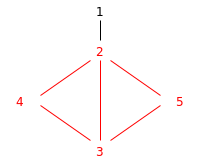Code Submission Evaluation System Login

# E4590 2018 1

 Start: 2018-09-15 13:00:00 End: 2018-09-15 16:00:00

CSES - E4590 2018 1 - Densest subgraphCSES - Densest subgraph

## Densest subgraph

 Time limit: 3.00 s Memory limit: 512 MB

Let $G = (V,E)$ be a simple, undirected graph; here $V$ is the set of nodes and $E$ is the set of edges.

The density of graph $G$ is $m/n$, where $m$ is the number of edges and $n$ is the number of nodes.

We define an induced subgraph as follows. Let $X \subseteq V$ be a nonempty subset of nodes. Then the subgraph induced by $X$, in notation $G[X]$, is formed by deleting all nodes that are not in $X$. Naturally, whenever you delete a node, you will have to delete all edges connected to it as well.

Given a graph $G = (V,E)$, your task is to find a set $X \subseteq V$ such that the density of $G[X]$ is as large as possible. We say that $G[X]$ is a densest subgraph of $G$.

Let us look at a simple example. Consider a graph $G$ with the set of nodes $V = \{1,2,3,4,5\}$ and that contains the edges $\{1,2\}$, $\{2,3\}$, $\{2,4\}$, $\{3,4\}$, $\{2,5\}$, and $\{3,5\}$. Now the density of $G$ is $6/5$. If you select e.g. $X = \{1,2\}$, you will have a subgraph $G[X]$ with only $1$ edge and $2$ nodes, so the density of $G[X]$ is only $1/2$; this is clearly a bad choice. However, if you select $X = \{2,3,4,5\}$, then you will have a subgraph $G[X]$ with $5$ edges and $4$ nodes; the density of $G[X]$ is $5/4$. This is the best possible choice in this case; there is no induced subgraph with a higher density.

Input

The first line contains two numbers, $n$ and $m$. This indicates that the input graph $G$ contains $n$ nodes and $m$ edges. The nodes are labelled $V = \{1,2,\dotsc,n\}$.

Then there are $m$ lines that list the edges. On each line there are two numbers, $u$ and $v$, with $u \ne v$ and $1 \le u,v \le n$. This indicates that there is an edge $\{u,v\} \in E$, that is, there is an undirected edge between the nodes $u$ and $v$ in graph $G$. Each edge is listed only once.

Output

Output a description of a densest subgraph $G[X]$. First, output a line with two numbers, $n'$ and $m'$, where $n' = |X|$ is the number of nodes in $G[X]$ and $m'$ is the number of edges in $G[X]$.

Then output one line with $n$ numbers: the labels of the nodes that are contained in subset $X$.

If there are multiple equally dense subgraphs, you can output any such solution.

Limits
• $2 \le n \le 100$
• $1 \le m \le \min(500, \frac{n(n-1)}{2})$
Example

Input:
5 6 1 2 2 3 2 4 3 4 2 5 3 5

Output:
4 5 2 3 4 5

Picture of the example:The nodes in the output and edges connecting them have been marked with red.